(Speed Mathematics)

People who excel at mathematics use better strategies than the rest of us, they don't necessarily have better brains. We teach simple strategies that can have you multiplying large numbers in your head, doing mental long division, even squaring and finding square roots of numbers off the top of your head.

And here is a secret. People equate intelligence with mathematical ability. In other words, if you are able to do lightning calculations in your head, people will think you are intelligent in other areas as well.

Here is one of my most important rules of mathematics. It is an unfair rule, but it is a rule just the same.

The easier the method you use to solve a problem, the faster you will solve it and there is less chance of making a mistake. The more complicated the method you use, the longer you take to solve it and greater the chance of making a mistake.

So, the people who use better methods are faster at getting the answer and make fewer mistakes. Those who use poor methods are slower getting the answer and make more mistakes. It doesn't have much to do with intelligence or having a "mathematical brain."

The methods we teach are not only fun to use, they are easy to learn. I walk into a class of elementary school children and teach them the basic multiplication tables in half an hour -- up to the twenty times tables. They are multiplying numbers like 95 times 95 in their heads faster than you can punch the numbers into a calculator. And the kids love it.
Engineers love showing off what they can do after attending a class.

The methods are more than techniques for fast calculation. They develop strategies for general problem solving. If you don't know , or haven't been taught how to solve a problem, you will work out your own method. Many students have already learnt these methods and developed reputations for being mathematical prodigies. Here is a short excerpt from some of my material on Easy Mathematics where I illustrate how an easy method can improve your ability.

MULTIPLICATION (PART ONE)

How well do you know your basic multiplication tables?

What would you think if I told you that you could master your tables up to the ten times table in less than 15 minutes? And your tables up to the twenty times table in less than half an hour? You can, using the methods we explain in this chapter. We only assume you know the two times table reasonably well, and that you can add and subtract simple numbers.

Let's go straight to the method. Here is how you multiply numbers up to ten times ten.

We'll take 7 X 8 as an example.

Write 7 X 8 = down on a piece of paper and draw a circle below each number to be multiplied.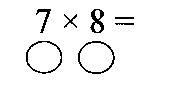Now go to the first number to be multiplied, 7. How many more do you need to make 10? the answer is three. Write 3 in the circle below the 7. Now go to the eight. What do we write in the circle below the eight? How many more to make 10? The answer is two. Write 2 in the circle below the eight. Your work should look like this.We now take away diagonally. Take either one of the circled numbers (3 or 2) away from the number, not directly above, but diagonally above, or crossways. In other words, you either take 3 from 8 or 2 from 7. Either way, the answer is the same, 5. This is the first digit of your answer. You only take away one time, so choose the subtraction you find easier. Now you multiply the numbers in the circles. 3 times 2 is 6. This is the last digit of your answer. The answer is 56. This is how the completed sum looks.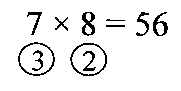Let's try another, 8 times 9.How many more to make ten? The answer is 2 and 1. We write 2 and 1 in the circles below the numbers. What do we do now? We take away diagonally. 8 - 1 = 7 or 9 - 2 = 7. 7 is the first digit of your answer. Write it down. Now multiply the two circled numbers together. 2 X 1 = 2, the last digit of the answer. The answer is 72. Isn't that easy? Here are some problems to try by yourself.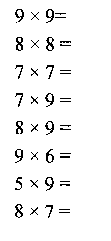Do all of the problems, even if you know your tables well. This is the basic strategy we will use for almost all of our multiplication.

How did you go? The answers are 81, 64, 49, 63, 72, 54, 45 and 56. Isn't this much easier than chanting your tables for 15 minutes every school day?

Does this method work for multiplying large numbers? It certainly does. Let's try it for 96 times 97.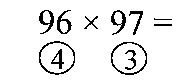What do we take these numbers up to? How many more to make what? One hundred. So we write 4 under 96 and 3 under 97.

What do we do now? We take away diagonally. 96 minus 3 or 97 minus 4 equals 93. Write that down as the first part of your answer. What do we do next? Multiply the numbers in the circles. 4 times 3 equals 12. Write this down for the last part of the answer. The full answer is 9,312.

Which method is easier, this method or the method you learnt in school? This method, definitely, don't you agree. Here is my first law of mathematics:

The easier the method you use, the faster you do the problem and the less likely you are to make a mistake. Now, here are some more problems to do by yourself.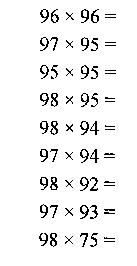The answers are 9,216, 9,215, 9,025, 9,310, 9,212, 9,118, 9,016, 9,021, 7,350. In the last problem, I hope you remembered to take 75 from a hundred, not eighty. Did you get them all right? If you made a mistake, go back and find where you went wrong and do it again.

That was an illustration of how easy these methods are to use. Students see instant results, so are motivated to try more.

Do these methods replace the need for learning tables? Not at all. They replace the method of learning tables. Typically, children can master their tables up to 20 times 20 in half an hour, and perform a lightning calculation to get an immediate answer. After calculating 7 times 8 equals 56, or 13 times 14 equals 182 maybe two dozen times, they start calling the answers from memory. Often they will do the calculation as they call the answer from memory just to "double check."

Does the method work for all numbers? Yes, it is based on a valid mathematical formula. What about multiplying numbers like 37 times 62? How about trying to multiply numbers like 6 times 7 or 3 times 7? Will the method work for multiplying numbers further apart like 97 times 645? How about using the strategies in reverse to divide? The methods will work to multiply any numbers; each variation of the formula is used to teach another principle of mathematics. Finding the easiest method will improve a student's ability to work with numbers and solve problems. Also, the methods help develop many other related number skills apart from multiplication.

The methods don't conflict with what schools teach, they support what the schools are doing. Many teachers around the world are now using these strategies to teach mathematics to their students.

The methods encourage creative thinking, and finding creative solutions to problems rather than using rules they have learned by rote. The methods encourage a better understanding of basic mathematical principles; students apply principles they already know in ways they had never dreamed possible.

Important Note

I have received many enquiries saying that the above formula doesn't work for all numbers. They say it doesn't work for 6 times 7 or 6 times 6. It does. I haven't given the full explanation on this web page. The formula does work for those numbers. For a full explanation, please purchase any of our mathematics programs.

SPEED MATHEMATICS volume 1 \$79.95Two extra long-play cassettes and workbooks enable you to master multiplication tables in less than half an hour, and be multiplying numbers like 96 X 97 in your head. It covers division, adding and subtracting, squaring and finding the square root of numbers mentally, and also how to check the answers. This program is suitable for grade 2 up to engineering level. Fun to use.

SPEED MATHEMATICS volume 2 \$79.95, or both 1 & 2, \$149.95Continues from volume one. A creative look at multiplication, squaring, long division by prime numbers using factors, simplified long division, problem solving.

Order the full program now.
LEARNING UNLIMITED AUSTRALIA P/L
(A.C.N. 006 433 629)
P.O. Box 545, Lilydale Vic. 3140, Australia

or order by credit card on (03) 9725 9756
or from North America, 011 613 9725 9756 (after 4.00 p.m. Pacific time.)

Fax (03) 9723 8401 in Australia or 011 613 9723 8401 in North America. (24 hours)

Order online with PayPalPrint order form
mailto:bhandley@virtual.net.au

• Maths Package - Special Offer
• Speed Maths For Kids - New Release
• Information on book, Speed Mathematics - Secrets of Lightning Calculation
• Information on book, Teach Your Children Tables
• Mathematics Package for teachers